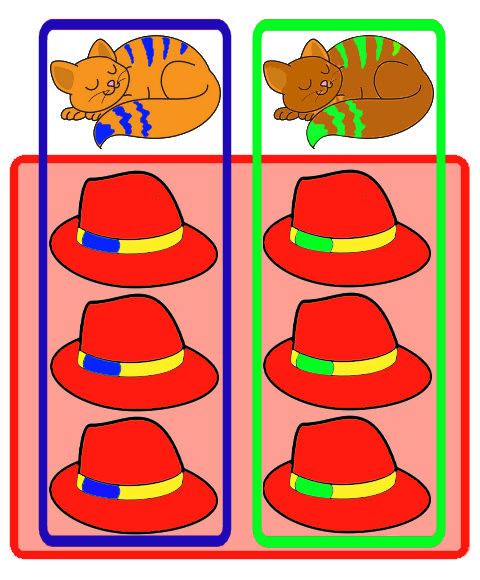Words Used
Before starting to learn your times tables, you must first learn some key words.
You should also understand what happens to numbers when you multiply and divide them.
Multiplication Division
X ÷
Multiplied Divided
Multiply Divide by
Times Shared by
Lots of Put into piles of
Groups ofIn this example, there are 2 cats and each has 3 hats. If you add (+) up all of the hats, there are six hats. If you want to know the total number of hats you need to multiply the number of cats by the number of hats each has. 2 cats multiplied by 3 hats equals 6 hats in total. All of these number facts are the same: 2 x 3 = 6 2 multiplied by 3 is 6 2 times 3 is 6 2 lots of 3 is 6 2 groups of 3 is 6 In multiplication, the two numbers being multiplied can swap places. This is called "commutative law." There are 2 cats. Each has 3 hats. In total there are 6 hats. (2 x 3 = 6) Is the same as: There are 3 hats that are given to each cat. There are 2 cats. In total there are 6 hats. (3 x 2 = 6)In this example, there are 2 cats and each has 3 hats. If you add (+) up all of the hats, there are six hats. If you want to know how many hats each cat gets when the six hats are shared equally, you need to divide the number of hats by the number of cats. 6 hats divided by 2 cats equals 3 hats per cat. All of these number facts are the same: 6 ÷ 2 = 3 6 divided by 2 is 3 6 shared by 2 is 3 6 put into piles of 2 is 3 In division, the numbers can NOT swap places.
Ask your teacher for the A4 times table number facts sheet so you can begin learning the number facts
If you need to print one at home click the button to open the pdf version.

Click on the button above for the section you want, and again to see the other options.

Home